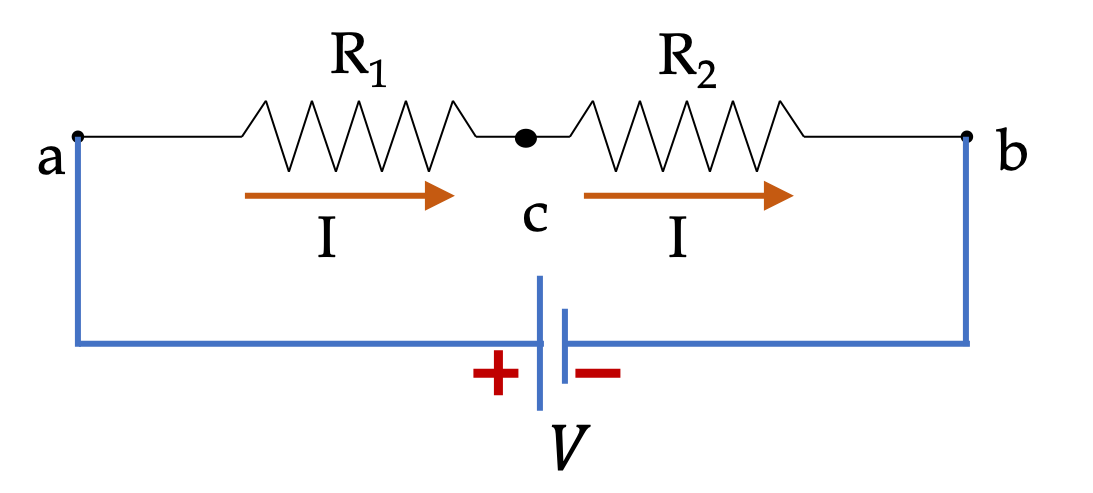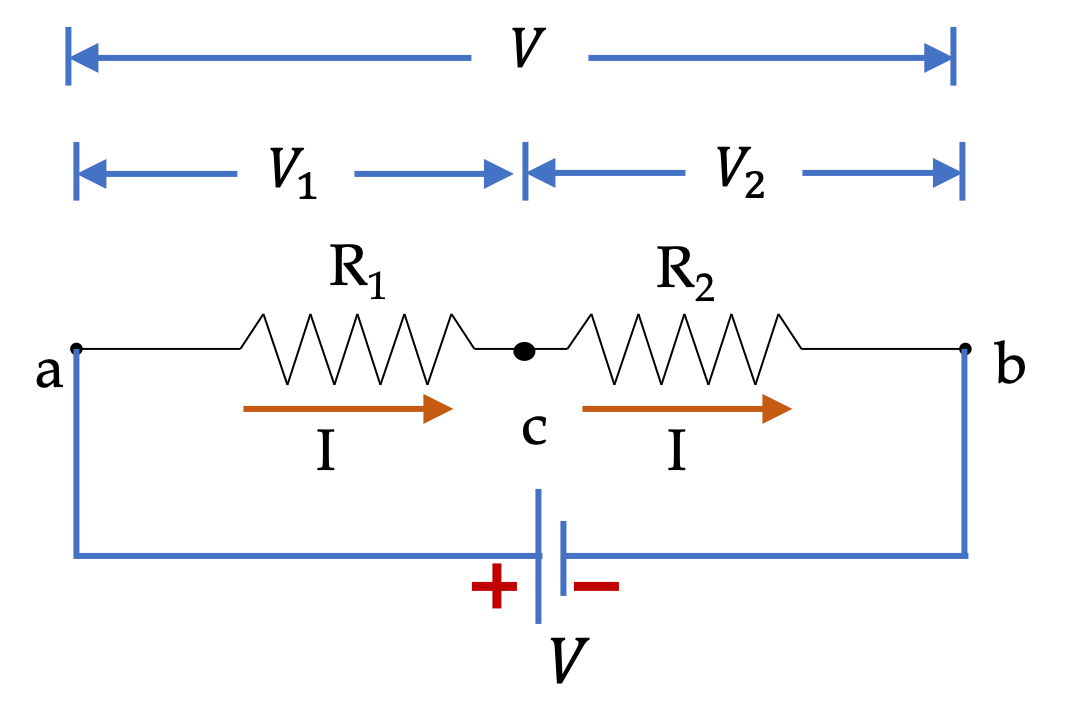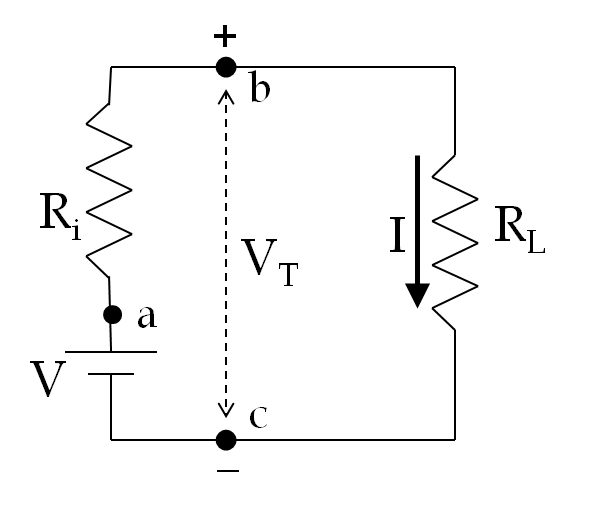## Section36.2Resistors in Series

When two resistors are connected end-to-end such that the same current must flow through both, then we say that the resistors are conected in series as shown in Figure 36.2.1.Figure 36.2.1. Two resitors in series in a circuit if same current passes through them both.

### Subsection36.2.1Current and Voltages in Series

In Figure 36.2.1, the resitors in series are connected to a voltage source such that we set up a potential differece across the two resistors.

\begin{equation} \phi_a - \phi_b = V.\label{eq-over-all-potential-drop-R-in-series-1}\tag{36.2.1} \end{equation}

But since we do not know the potential drop across either of the two resitors, we cannot apply Ohm's law to either resitor to find the current through them. If we apply Ohm's law to each of the two resitors we will get the following equations with unknown $\phi_c$ as well as $I\text{.}$

\begin{align} \phi_a - \phi_c \amp = I R_1. \label{eq-ohms-law-for-R1}\tag{36.2.2}\\ \phi_c - \phi_b \amp = I R_2. \label{eq-ohms-law-for-R2}\tag{36.2.3} \end{align}

We can think of finding $I$ as an algebra problem for these two equations. You can eliminate $\phi_c$ by adding the two equations and solve for $I\text{.}$

\begin{equation} I = \dfrac{V}{R_1 + R_2}.\tag{36.2.4} \end{equation}

Using this in the two equations above, we can immediately find the voltage drops across each resitor.

\begin{align*} \Delta\phi_1 \amp = \phi_a - \phi_c = V \dfrac{ R_1 }{R_1 + R_2}. \\ \Delta\phi_2 \amp =\phi_c - \phi_b = V \dfrac{ R_2 }{R_1 + R_2}. \end{align*}

From these you can see that total voltage drop $V$ has been proportionaltely shared by the two resistors in series. We say that resitors in series “divide-up” the total voltage acoss them in proportion of their own resitance - the resitor with higher resitance has the higher proportion of voltage across it.

An open circuit with no connection, say between $b$ and $c$ in Figure 36.2.1 will have $R_2=\infty\text{,}$ and all voltage will drop across such an open line. We call such places in a circuit to be open circuit.

### Subsection36.2.2Equivalent Resistance of Resitors in Series

It is instructive to think of resistors in series conceptually, as well, which we do next. To find the common current $I$ through resistors in series, we will combine them into one resistor, to which we can apply Ohm's law. For instance, if resistor $R_1$ is a $5\text{-m}$ copper wire and $R_2$ is a $3\text{-m}$ copper wire, then we can replace them with $5+3=8\text{-m}$ wire of same thickness. That means, the net resitance of two resistors in series is

\begin{equation*} R_\text{series} = R_1 + R_2. \end{equation*}

These arguments for two resitors in series can be easily extend to the case of $N$ resistors in series, giving us

\begin{equation} R_\text{series} = R_1 + R_2 + \cdots + R_N.\tag{36.2.5} \end{equation}

We say that between points $a$ and $b\text{,}$ in Figure 36.2.1 the two resistors present an equivalent resistance, $R_\text{equiv}\text{,}$

\begin{equation*} R_\text{equiv} = R_\text{series} = R_1 + R_2. \end{equation*}

We may think of an equivalent circuit that has only one resitor, with resistance $R_\text{equiv}$ rather than two resistors of the original circuit, without affecting the original circuit outside of points $a$ and $b$, as indicated in Figure 36.2.2.

Now, we can apply Ohm's law for this equivalent resistor to obtain current $I$ as

\begin{equation} I = \dfrac{\Delta \phi}{R_\text{equiv}} = \dfrac{\phi_a - \phi_b}{R_1 + R_2} = \dfrac{V}{R_1 + R_2},\tag{36.2.6} \end{equation}

where I have replaced $\phi_a-\phi_b$ by $V$ as we noted in Eq. (36.2.1).Figure 36.2.2. Two resitors in series in a circuit can be replaced by an equivalent resistor without affecting circuit outside of points $a$ and $b\text{.}$

### Subsection36.2.3Potential Drops Across Each Resistor in Series

Now, we can use Ohm's law on each of the resistor, as in Eqs. (36.2.2) and (36.2.3), to find potential drops across each.

\begin{align} \Delta\phi_1 = \phi_a - \phi_c \amp = \dfrac{R_1}{R_1 + R_2}\, V, \label{eq-ohms-law-for-R1-potential-diff}\tag{36.2.7}\\ \Delta\phi_2 = \phi_c - \phi_b \amp = \dfrac{R_2}{R_1 + R_2}\, V. \label{eq-ohms-law-for-R2-potential-diff}\tag{36.2.8} \end{align}

An important conceptual lesson to note here is that total potential drop across the two resistors, $\Delta\phi = \phi_a - \phi_b\equiv V\text{,}$ is actually a sum of the potential drops $\Delta\phi_1 = \phi_a - \phi_c\equiv V_1$ and $\Delta\phi_2 = \phi_c - \phi_b\equiv V_2$ across the two resitors in series.

\begin{equation*} V = V_1 + V_2. \end{equation*}We say that original voltage across the two has been divided up between the two resitors. We say that resitors in series is a volatage-divider circuit! Eqs. (36.2.7) and (36.2.8) tell us that the total voltage drop $V$ has been proportionaltely shared by the two resistors in series. We say that resitors in series “divide-up” the total voltage acoss them in proportion of their own resitance - the resitor with higher resitance has the higher proportion of voltage across it.

The circuit in Figure 36.2.4 shows two resitors in series with a voltage source.

(a) Find current through each resitor.

(b) Find voltage across each resitor.

(c) Find power dissipated in each resistor.

(d) How much energy is delivered to the circuit by the voltage source in $10\text{ minutes}\text{.}$

Hint

(a)-(c) Use definitions. (d) Use energy conservation.

(a) $0.4\text{ A}\text{,}$ (b) $4\text{ V}\text{,}$ $8\text{ V}\text{,}$ (c) $1.6\text{ W} \text{,}$ $3.2\text{ W} \text{,}$ (d) $2,880\text{ J} \text{.}$

Solution 1 (a)

(a) Since the resitors are in series, same current $I$ flows through both resitors and encounters a net resistance $R_1 + R_2\text{.}$

\begin{align*} I \amp = \dfrac{V}{R_1 + R_2} = \dfrac{12\ \text{V}} {10\ \Omega + 20\ \Omega} = 0.4\text{ A}. \end{align*}
Solution 2 (b)

(b) Same current $I$ flows through both resitors. Applying Ohm's law on each resitor will give us the potential drops across each of the resitors.

\begin{align*} V_1 \amp = I_1R_1 = IR_1 = 0.4\text{ A} \times 10\ \Omega = 4\text{ V},\\ V_2 \amp = I_2R_2 = IR_2 = 0.4\text{ A} \times 20\ \Omega = 8\text{ V}. \end{align*}
Solution 3 (c)

(c) Power dissipated in a resitor equals $IV$ for that resistor.

\begin{align*} P_1 \amp = I_1 V_1 = I V_1 = 0.4\text{ A} \times 4\text{ V} = 1.6\text{ W}.\\ P_2 \amp = I_2 V_2 = I V_1 = 0.4\text{ A} \times 8\text{ V} = 3.2\text{ W}. \end{align*}
Solution 4 (d)

(d) Energy conservation means the energy lost to the resitors must be suplied by the voltage source. Therefore, the net power of the source must be

\begin{equation*} P_\text{source} = P_1 + P_2 = 4.8\text{ W}. \end{equation*}

Therefore energy supplied in $10\text{ minutes}$ will be

\begin{equation*} E = P_\text{source}\Delta t = 4.8\text{ W} \times 600\text{ s} = 2,880\text{ J}. \end{equation*}

Every battery has an internal resistance. As a result voltage measured at the terminals is not equal to the EMF of the battery. While EMF of the battery is determined by the chemical potential of the chemicals involved, the potential difference, i.e., the voltage across terminals, depends on the resistance of the load connected across the terminals as we will show in the solution here.

If you want to explore the issue yourself, try finding a formula for the potential drop across the terminals in the following figure, which has a battery with EMF $V$ and internal resistance $R_i$ connected to a load of resistance $R_L\text{.}$ Then, explore $V_T$ as a function of $R_L\text{.}$ What happens when $R_L \gt\gt R_i\text{?}$Hint

$R_i$ and $R_L$ are in series.

$V_T = \dfrac{R_L}{R_L + R_i}\, V \text{.}$

Solution

From the circuit, we see that $R_L$ and $R_i$ are in series. We also see that the terminal voltage is just the potential drop across the load $R_L\text{.}$ Therefore,

\begin{equation*} V_T = \phi_b - \phi_c = I R_L = \dfrac{R_L}{R_L + R_i}\, V. \end{equation*}

Therefore, the voltage across the terminals of a battery depends upon whatever you connect across its terminals. However, if $R_i \lt \lt R_L\text{,}$ then $V_t$ can be approximately independent of the load.

\begin{equation*} V_T = \dfrac{R_L}{R_L + R_i}\, V \approx V\text{ if }R_i=0\text { or } R_i \lt\lt R_L. \end{equation*}

This can be illustrated for a voltaic cell of EMF 1.5 volts and internal resistance $1\ \Omega\text{.}$ With a $1000\text{-} \Omega$ resistor load, the terminal voltage will be approximately 1.5 volts, but with a $0.5\text{-}\Omega$ load resistor, the voltage across the same terminals will be only 0.5 volt!